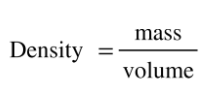# Problem: Calculate these volumes.(a) What is the volume of 25 g iodine, density = 4.93 g/cm3 ?

###### FREE Expert Solution
• Density can be calculated as:###### Problem Details

Calculate these volumes.

(a) What is the volume of 25 g iodine, density = 4.93 g/cm3